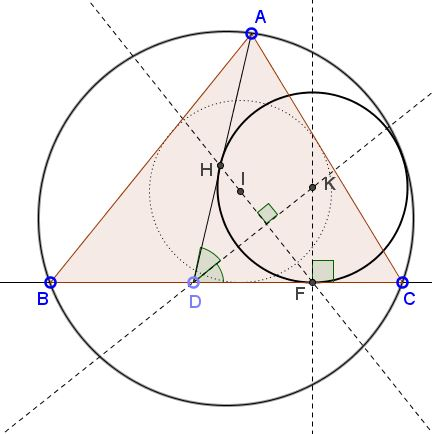# Construction of Thébault Circles

### Problem

Given $\Delta ABC$ and point $D$ on $BC.$Construct a circle tangent to $AD,$ $CD,$ and the circumcircle of $\Delta ABC.$

### Solution

Solution proceeds in several steps:1. Find the incenter $I$ of $\Delta ABC.$

2. Drop a perpendicular from $I$ to the bisector of $\angle ADC.$ Mark its intersection with $BC$ - $F.$

3. p>Erect a perpendicular to $BC$ at $F$. Mark $K$ - its intersection with the bisector of $\angle ADC.$

$K$ is the center of Thébault's circle.

For a proof, recollect Y. Sawayama's Lemma: If $F$ is the point of tangency of the Thébault circle $(K)$ and $BC$ then $IF$ passes through its point of tangency with $AD,$ say $H.$ But $FH$ is perpendicular to the bisector of $\angle ADC$ into which $(K)$ is inscribed.

### Thébault's Problems

• Thébault's Problem I
• Thébault's Problem II
• Thébault's Problem III
• Y. Sawayama's Lemma
• Jack D'Aurizio Proof of Sawayama's Lemma
• Y. Sawayama's Theorem
• Thébault's Problem III, Proof (J.-L. Ayme)
• Circles Tangent to Circumcircle
• Thébault's Problem IV
• A Property of Right Trapezoids
• A Lemma on the Road to Sawayama
• Excircles Variant of Thébault's Problem III
• In the Spirit of Thebault I
• Dao's Variant of Thebault's First Problem
•